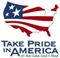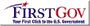Data Series 283

U.S. GEOLOGICAL SURVEY
Data Series 283

## Inch/Pound to SI

Multiply By To obtain
Length
inch (in.) 2.54 centimeter (cm)
foot (ft) 0.3048 meter (m)
mile (mi) 1.609 kilometer (km)
Area
acre 4,047 square meter (m2)
Volume
quart (qt) 0.9464 liter (L)
Flow rate
gallon per minute (gal/min) 0.06309 liter per second (L/s)
Mass
pound, avoirdupois (lb) 0.4536 kilogram (kg)
picocurie per liter (pCi/L) 0.037 becquerel per liter (Bq/L)

Temperature in degrees Celsius (°C) may be converted to degrees Fahrenheit (°F) as follows:

°F=(1.8×°C)+32

Altitude, as used in this report, refers to distance above the National Geodetic Vertical Datum of 1929 (NGVD29).

Horizontal coordinate information is referenced to the North American Datum of 1983 (NAD83).

Specific conductance is given in microsiemens per centimeter at 25 degrees Celsius (µS/cm at 25°C).

Concentrations of chemical constituents in water are given either in milligrams per liter (mg/L) or micrograms per liter (µg/L).

## Abbreviations

meq/L milliequivalents per liter
per mil parts per thousandU.S. Department of the Interior | U.S. Geological Survey